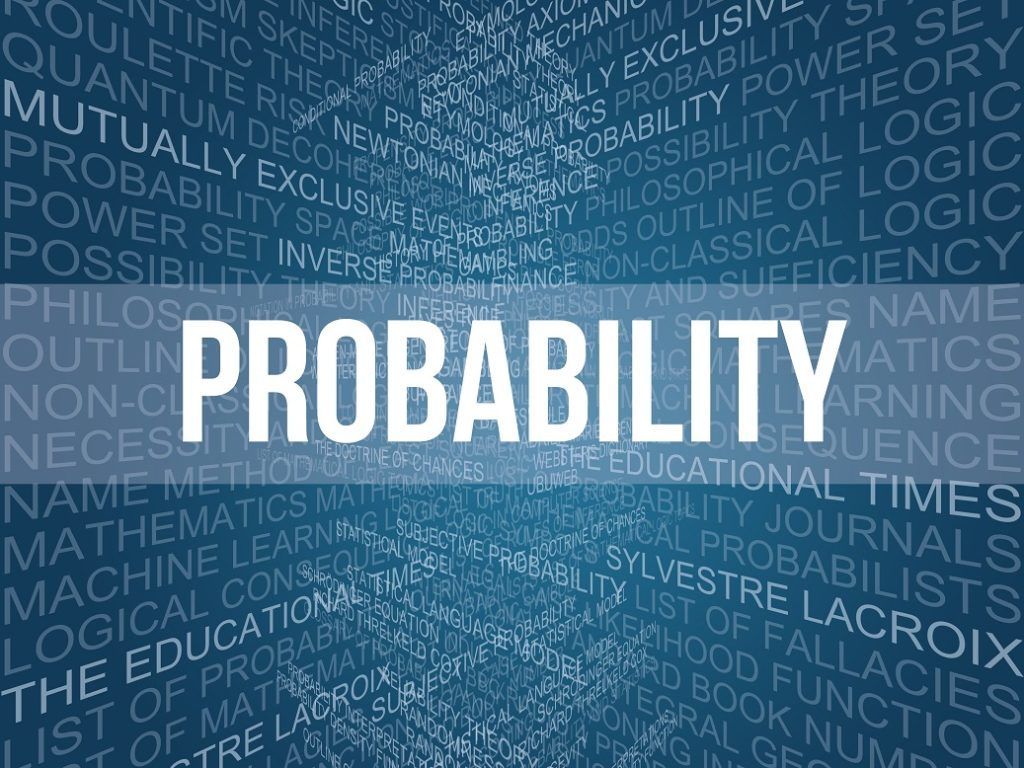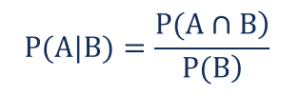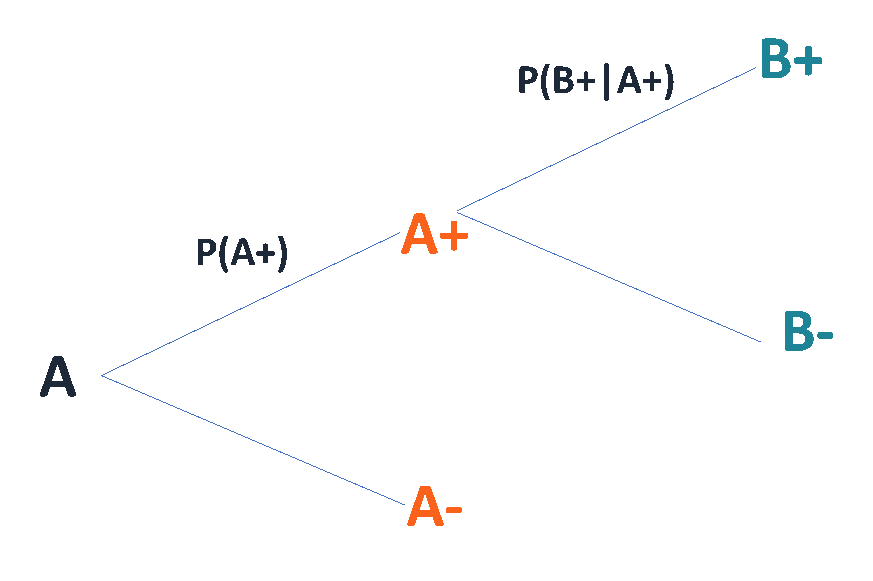# Conditional Probability

The probability of an event occurring given that the other event has already occurred

## What is Conditional Probability?

Conditional probability is the probability of an event occurring given that the other event has already occurred. The concept is one of the quintessential concepts in probability theory. Note that the conditional probability does not state that there is always a causal relationship between the two events, as well as it does not indicate that both events occur simultaneously.Also, the concept of conditional probability is primarily related to the Bayes’ theorem, which is one of the most influential theories in statistics.

### Formula for Conditional ProbabilityWhere:

• P(A|B) – the conditional probability; the probability of event A occurring given that event B has already occurred
• P(A ∩ B) – the joint probability of events A and B; the probability that both events A and B occur at the same time
• P(B) – the probability of event B

The formula above is applied to the calculation of the conditional probability of events that are neither independent nor mutually exclusive.

Another way of calculating conditional probability is by using the Bayes’ theorem. The theorem can be used to determine the conditional probability of event A, given that the event B has occurred by knowing the conditional probability of event B, given the event A has occurred, as well as the individual probabilities of the event A and B. Mathematically, the Bayes’ theorem can be denoted in the following way:Finally, conditional probabilities can be found using a tree diagram. In the tree diagram, the probabilities in each branch are conditional.### Conditional Probability for Independent Events

Two events are independent if the probability of the outcome of one event does not influence the probability of the outcome of another event. Due to this reason, the conditional probability of two independent events A and B is:

### Conditional Probability for Mutually Exclusive Events

In probability theory, mutually exclusive events are the events that cannot occur simultaneously. In other words, if one event has already occurred, another can event cannot occur. Thus, the conditional probability of the mutually exclusive events is always zero.